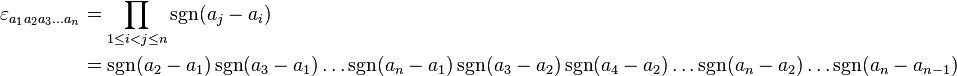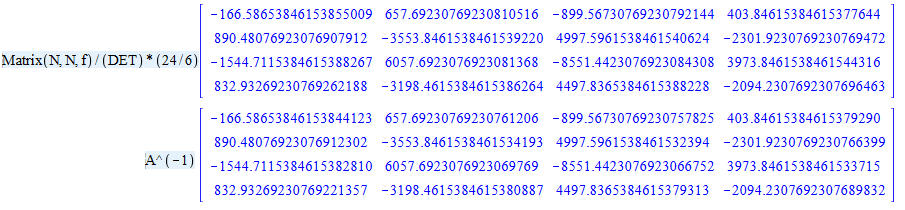# Question:Levi-Civita symbol and inverse of matrix

## Question:Levi-Civita symbol and inverse of matrix

Maple

Hi

We know determinant of a square matrix A[ij] (i,j ∈ {1,2,3}) is equal to the following expression

det(A) = 1/6 * e[ijk] * e[pqr] * A[ip] * A[jq] * A[kr]

in which e[ijk] is a third order Tensor (Permutation notation or Levi-Civita symbol) and has a simple form as follows:

e[mnr] = 1/2 * (m-n) * (n-r) * (r-m).

The (i,j) minor of A, denoted Mij, is the determinant of the (n − 1)×(n − 1) matrix that results from deleting row i and column j of A. The cofactor matrix of A is the n×n matrix C whose (i, j) entry is the (i, j) cofactor of A,

C[ij]= -1 i+j * M[ij]

A-1=CT/det(A)

The general form of Levi-Civita symbol is as bellow:I want to write a program for finding inverse of (NxN) matrix:

N=2 →

restart;
N := 2:
with(LinearAlgebra):
f := (1/2)*(sum(sum(sum(sum((m-n)*(p-q)*A[m, p]*A[n, q], q = 1 .. 2), p = 1 .. 2), n = 1 .. 2), m = 1 .. 2)):
A := Matrix(N, N, proc (i, j) options operator, arrow; evalf((37*i^2+j^3)/(2*i+4*j)) end proc):
f/Determinant(A);

N=3 →

restart;
N := 3:
with(LinearAlgebra):
f := (1/24)*(sum(sum(sum(sum(sum(sum((m-n)*(n-r)*(r-m)*(p-q)*(q-z)*(z-p)*A[m, p]*A[n, q]*A[r, z], m = 1 .. N), n = 1 .. N), r = 1 .. N), p = 1 .. N), q = 1 .. N), z = 1 .. N)):
A := Matrix(N, N, proc (i, j) options operator, arrow; 10*i^2/(20*i+j) end proc):
f/Determinant(A);

The results of above programs are equal to 1 and validation of method is observed.

If we can write the general form of determinant then we can find the inverse of any square non-singular matrices.

Now I try to write the mentioned program.

restart;
with(linalg):
N := 7:
Digits := 40:
e := product(product(signum(a[j]-a[i]), j = i+1 .. N), i = 1 .. N):
ML := product(A[a[k], b[k]], k = 1 .. N):
s := e*subs(`\$`(a[q] = b[q], q = 1 .. N), e)*ML:
for i to N do
s[i] := sum(sum(s[i-1], a[i] = 1 .. N), b[i] = 1 .. N)
end do:
A := Matrix(N, N, proc (i, j) options operator, arrow; evalf((3*i+j)/(i+2*j)) end proc): # arbitrary matrix
CN:=simplify(s[N]/det(A));

Therefore det(A)= CN-1 * e[a1,a2,..an] * e [b1,b2,.., bn] * A[a1,b1] * A[a2,b2] * ... * A[an,bn].

The correction coefficient is CN(for N)/CN(for N-1) = N!/(N-1)! =N.

restart:
with(linalg): N := 4: Digits := 20:
e := product(product(signum(a[j]-a[i]), j = i+1 .. N), i = 1 .. N):
ML := product(A[a[k], b[k]], k = 1 .. N):
s := e*subs(`\$`(a[q] = b[q], q = 1 .. N), e)*ML:
for r to N do s[r] := sum(sum(s[r-1], a[r] = 1 .. N), b[r] = 1 .. N) end do:
A := Matrix(N, N, proc (i, j) options operator, arrow; evalf((3*i+2*j)/(i+2*j)) end proc):
DET:=S[N]:
for x to N do for y to N do
e := product(product(signum(a[j]-a[i]), j = i+1 .. N-1), i = 1 .. N-1):
ML := product(AA[a[k], b[k]], k = 1 .. N-1):
S[0, x, y] := e*subs(`\$`(a[q] = b[q], q = 1 .. N-1), e)*ML:
for r to N-1 do S[r, x, y] := sum(sum(S[r-1, x, y], a[r] = 1 .. N-1), b[r] = 1 .. N-1) end do:
f[y, x] := (-1)^(x+y)*subs(seq(seq(AA[t, u] = delrows(delcols(A, y .. y), x .. x)[t, u], t = 1 .. N-1), u = 1 .. N-1), S[N-1, x, y])
end do: end do:
Matrix(N, N, f)/(DET)*(24/6);
A^(-1);CN for N=4 and N=3 is 24 and 6 respectively i.e. CN(4)/CN(3)=24/6.

When I use bellow procedure the error "(in S) bad index into Matrix" is occurred.

Thank you

restart; with(linalg): Digits := 40: n := 7:
S := proc (N) local e, ML, s, i:
e := product(product(signum(a[j]-a[i]), j = i+1 .. N), i = 1 .. N):
ML := product(A[a[k], b[k]], k = 1 .. N):
s := e*subs(`\$`(a[q] = b[q], q = 1 .. N), e)*ML:
for i to N do s[i] := sum(sum(s[i-1], a[i] = 1 .. N), b[i] = 1 .. N) end do
end proc:
A := Matrix(n, n, proc (i, j) options operator, arrow; evalf((3*i+j)/(i+2*j)) end proc): # arbitrary matrix
CN := simplify(S(n)/det(A))﻿